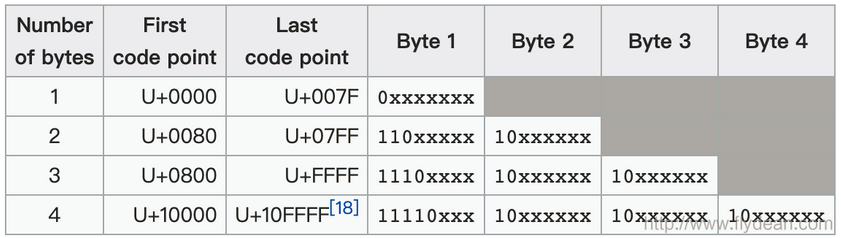# java安全编码指南之:字符串和编码

0收藏

char不能表示所有的Unicode

StringBuilder和StringBuffer还是使用的是char[]。UTF-8使用1到4个字节表示对应的字符，而UTF-16使用2个或者4个字节来表示对应的字符。

``````public String readByteWrong(InputStream inputStream) throws IOException {
byte[] data = new byte;
int offset = 0;
String str="";

str += new String(data, offset, bytesRead, "UTF-8");
if (offset >= data.length) {
throw new IOException("Too much input");
}
}
return str;
}``````

`````` public String readByteCorrect(InputStream inputStream) throws IOException {
char[] data = new char;
int offset = 0;
String str="";

str += new String(data, offset, charRead);
if (offset >= data.length) {
throw new IOException("Too much input");
}
}
return str;
}``````

char不能表示所有的Unicode

``````public static String subStringWrong(String string) {
char ch;
int i;
for (i = 0; i < string.length(); i += 1) {
ch = string.charAt(i);
if (!Character.isLetter(ch)) {
break;
}
}
return string.substring(i);
}``````

``````public static String subStringCorrect(String string) {
int ch;
int i;
for (i = 0; i < string.length(); i += Character.charCount(ch)) {
ch = string.codePointAt(i);
if (!Character.isLetter(ch)) {
break;
}
}
return string.substring(i);
}``````

``````public void toUpperCaseWrong(String input){
if(input.toUpperCase().equals("JOKER")){
System.out.println("match!");
}
}``````

``````public void toUpperCaseRight(String input){
if(input.toUpperCase(Locale.ENGLISH).equals("JOKER")){
System.out.println("match!");
}
}``````

``````    public void getDateInstanceWrong(Date date){
String myString = DateFormat.getDateInstance().format(date);
}

public void getDateInstanceRight(Date date){
String myString = DateFormat.getDateInstance(DateFormat.MEDIUM, Locale.US).format(date);
}​``````

``````   public void fileOperationWrong(String inputFile,String outputFile) throws IOException {
PrintWriter writer = new PrintWriter(new FileWriter(outputFile));
int line = 0;
line++;
}
writer.close();
}``````

``````

PrintWriter writer = new PrintWriter(new OutputStreamWriter(new FileOutputStream(outputFile), Charset.forName("UTF8")));​``````

``````    public void convertBigIntegerWrong(){
BigInteger x = new BigInteger("1234567891011");
System.out.println(x);
byte[] byteArray = x.toByteArray();
String s = new String(byteArray);
byteArray = s.getBytes();
x = new BigInteger(byteArray);
System.out.println(x);
}``````

1234567891011
80908592843917379

``````public void convertBigIntegerRight(){
BigInteger x = new BigInteger("1234567891011");
String s = x.toString();  //转换成为可以存储的字符串
byte[] byteArray = s.getBytes();
String ns = new String(byteArray);
x = new BigInteger(ns);
System.out.println(x);
}``````

``````  public void convertBigIntegerWithBase64(){
BigInteger x = new BigInteger("1234567891011");
byte[] byteArray = x.toByteArray();
String s = Base64.getEncoder().encodeToString(byteArray);
byteArray = Base64.getDecoder().decode(s);
x = new BigInteger(byteArray);
System.out.println(x);

}``````

learn-java-base-9-to-20/tree/master/security